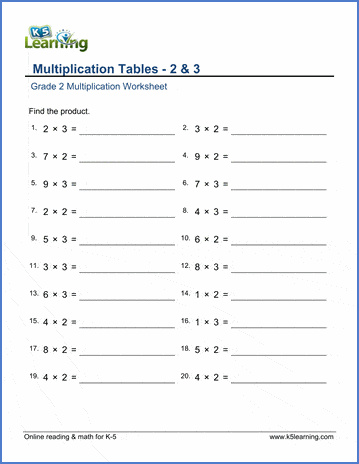# 2nd Grade Math Worksheets: Multiplication

These grade 2 multiplication worksheets emphasize early multiplication skills; in particular recall of the 2, 5 and 10 'times tables', multiplying by whole tens and solving missing factor problems.

## Meaning of multiplication

Meaning of multiplication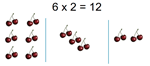Multiplication sentences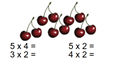Rows, columns & arrays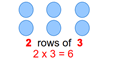Multiplication with arrays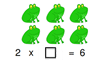## Multiplication tables

Multiplication tables of 2 and 3 2 x 5 =
Multiplication tables of 5 and 10 5 x 9 =
Tables 2-5 practice 2 x 4 =
Tables 2, 5, 10 practice 5 x 2 =

## Missing factors

Table of 2 - missing factor 2 x __ = 18
Table of 5 - missing factor 5 x __ = 15
Table of 10 - missing factor 10 x __ = 100
Tables 2, 5, 10 - missing factor __ x 10 = 20

## Multiplying by 2

Two times small numbers 2 x 14 =
Two times multiples of 5 2 x 55 =
Two times whole tens 2 x 70 =
Two times whole tens, missing factor ___ x 90 = 180

## Multiplication word problems

Multiplication word problems (within 25)